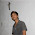# How to convert String to int in Java

There are 3 main ways to convert String to int in Java, using the constructor of Integer class, parseInt() method of java.lang.Integer and Integer.valueOf() method. Though all those methods return an instance of java.lang.Integer, which is a wrapper class for primitive int value, it's easy to convert Integer to int in Java. From Java 5, you don't need to do anything, autoboxing will automatically convert Integer to int. For Java 1.4 or lower version, you can use intValue() method from java.lang.Integer class, to convert Integer to int. As the name suggest, parseInt() is the core method to convert String to int in Java. parseInt() accept a String which must contain decimal digits and first character can be an ASCII minus sign (-) to denote negative integers. parseInt() throws NumberFormatException, if provided String is not convertible to int value.

By the way parseInt is an overloaded method and it's overloaded version takes radix or base e.g. 2,8,10 or 16, which can be used to convert binary, octal, hexadecimal String to int in Java. Integer.valueOf() is another useful method to convert String to Integer in Java, it offers caching of Integers from -128 to 127.

Internally, valueOf() also calls parseInt() method for String to int conversion. In this Java programming tutorial, we will see all three ways to convert String to int value in Java.

## String to Integer Conversion ExampleThis Java program converts both positive and negative numeric String to int in Java. As I said, it uses three methods. First example uses constructor of Integer class to create Integer from String, later that Integer is converted to int using autoboxing in Java

Second example uses Integer.parseInt() and third String conversion examples use Integer.valueOf() method to convert String to int in Java.

By the way, be careful with String.valueOf() method, as it may return same object on multiple class, if integer value is from it's cache. Also comparing int to Integer is error prone in autoboxing and can produce surprising results. See pitfalls of autoboxing in Java for more details.

```package test;

/**
* Java program to convert String to int in Java. From Java 5 onwards
* autoboxing can be used to convert Integer to int automatically.
*
* @author http://java67.blogspot.com
*/
public class StringToIntegerHowTo {

public static void main(String args[]) {

//Converting positive integer value String to int in Java
String integer = "123";

// String to int conversion using Integer constructor and autoboxing
int number = new Integer(integer);
System.out.println("Integer created from String in Java : " + number);

// Converting String to int using parseInt() method of java.lang.Integer
number = Integer.parseInt(integer);
System.out.println("String to int using parseInt()  : " + number);

// String to int conversion using valueOf() method of Integer class
number = Integer.valueOf(integer);
System.out.println("String to int Conversion example using valueOf  : " + number);

String negative = "-123";
System.out.println("Converting negative String to int in Java");
System.out.println("String to Integer Constructor Example : " + new Integer(negative));
System.out.println("String to Integer parseInt Example : " + Integer.parseInt(negative));
System.out.println("String Integer valueOf Example : " + Integer.valueOf(negative));

}

}

Output:
Integer created from String in Java : 123
String to int using parseInt()  : 123
String to int Conversion example using valueOf  : 123
Converting negative String to int in Java
String to Integer Constructor Example : -123
String to Integer parseInt Example : -123
String Integer valueOf Example : -123```

That's all on How to convert String to int or Integer in Java. By the way, converting int to Integer is ridiculously easy in Java and done automatically by Java platform, from Java 5 onwards. As said in best way to convert String to number in Java, you can choose between parseInt() and valueOf() for String to int conversion. Integer.valueOf() is preferred because it offers caching and eventually delegate call to parseInt for String to int conversion, if integer value is not available in cache.

Further Learning
Complete Java Masterclass
Java Fundamentals: The Java Language
Java In-Depth: Become a Complete Java Engineer!

Related Java Programming Tutorials for Java programmers

1.I cannot see the other codes in the right side

1.They are wrapped up into next line.

2.I was asked to write a method which takes String and return equivalent int value e.g. convert("1") should return 1. catch was I cannot use any method from Java API e.g. Integer.parseInt() or Integer.valueOf(). this was more like atoi() method of C, C++. does anyone knows solution in Java?

3.How to give input as string in java

1.@Anonymous, you can either use system property e.g. java -Dinput=yourname or you can write code to prompt user to input by using Scanner class. By default anything you enter is String in Java. I have already blogged about it here, you would like to see this post